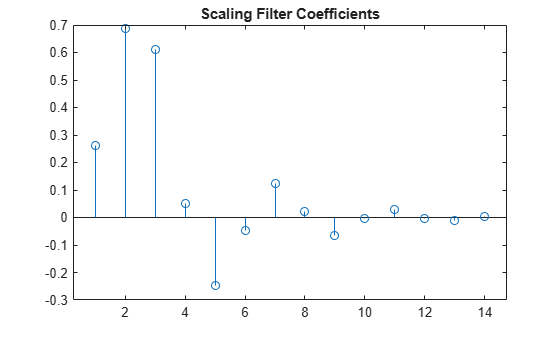# fejerkorovkin

Fejér-Korovkin wavelet filters

## Syntax

``Lo = fejerkorovkin(wname)``

## Description

example

````Lo = fejerkorovkin(wname)` returns the Fejér-Korovkin scaling filter specified by `wname`. Valid entries for `wname` are `'fk4'`, `'fk6'`, `'fk8'`, `'fk14'`, `'fk18'`, and `'fk22'`. For information on the Fejér–Korovkin filters, see Nielson.```

## Examples

collapse all

Construct and plot the Fejér-Korovkin (14) scaling function and wavelet.

Obtain the Fejér-Korovkin scaling filter and display its 14 coefficients.

`Lo = fejerkorovkin("fk14")`
```Lo = 1×14 0.2604 0.6869 0.6116 0.0514 -0.2456 -0.0486 0.1243 0.0222 -0.0640 -0.0051 0.0298 -0.0033 -0.0093 0.0035 ```

Use the scaling filter to obtain the wavelet filter and display its wavelet filter coefficients.

`Hi = qmf(Lo)`
```Hi = 1×14 0.0035 0.0093 -0.0033 -0.0298 -0.0051 0.0640 0.0222 -0.1243 -0.0486 0.2456 0.0514 -0.6116 0.6869 -0.2604 ```

`wavefun` provides an efficient way to construct and plot the scaling function and wavelet.

```[phi,psi,xval] = wavefun("fk14"); subplot(2,1,1) plot(xval,phi) title("Scaling Function") subplot(2,1,2) plot(xval,psi) title("Wavelet")```## Input Arguments

collapse all

Name of desired Fejér-Korovkin scaling filter. The numeric value in each name is the number of Fejér-Korovkin filter coefficients. For example, if `wname` is `'fk14'`, `Lo` has 14 coefficients.

## Output Arguments

collapse all

Scaling filter, returned as a vector.

 Nielsen, M. "On the construction and frequency localization of finite orthogonal quadrature filters." Journal of Approximation Theory. Vol. 108, Number 1, 2001, pp. 36–52.